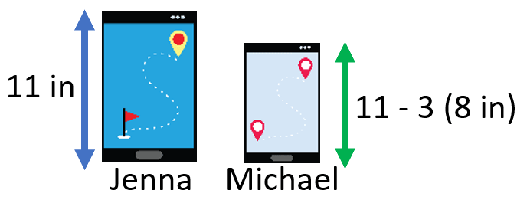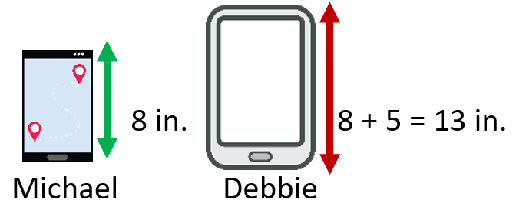# Math Worksheets Land

Math Worksheets For All Ages

# Math Worksheets Land

Math Worksheets For All Ages

# Length Word Problems Worksheets

These worksheets are pretty specific they include word problems that include not only length, but an addition or subtraction operation. How to Outline a Math Word Problem: -Solving a word problem is never an easy job. If you are also one of those who face difficulties solving math problems, then you must find out an easy solution way to solve them. An alternative could be outlining the problem to solve it. Outlines are more like strategies that can help you approach a complex problem systematically and easily. Learn how you can develop an outline for solving word problems.
- Read the problem carefully and identify necessary information that provides a clue to solving the problem (measurements, total/sum, difference). Segregate any extra or irrelevant information from the word problem. - Draw a table, diagram, or model to insert the relevant information into it. - Look for any patterns or trends in the problem - Now see what you need to find out in the problem. - You need to name mathematical symbols to identify what the problem says. For example; difference is a – (minus), sum is + (plus), product is x (multiplication) and division is / (divide). - Start to solve.

### Aligned Standard: Grade 2 Measurement - 2.MD.5

• Step-by-step Lesson- Work through sentence based problems to determine the length of a sum or difference of measures.
• Guided Lesson - Find out who has the longest or shortest bed, key, or school bus.
• Guided Lesson Explanation - I find it is best to explain the problems by determining the measure of all people in the story.
• Practice Worksheet - Ten sentence based problems for you to go through and compare.
• Matching Worksheet - Match the problem to the described value in each problem.
• Answer Keys - These are for all the unlocked materials above.

### Homework Sheets

We start off really simple with the help of visual prompts.

• Homework 1- Edna's doll is 15 centimeters tall. Alley's doll is 2 centimeters taller than Edna's. Joy's doll is 3 centimeters shorter than Edna's. Which doll is taller than 15 centimeters?
• Homework 2- Brooklyn's car is 8 feet long. Megan's car is 2 feet shorter than Brooklyn's. Who has the longer car?
• Homework 3- Vanessa's cycle is 5 meters long. Evelyn's cycle is 1 meter shorter than Vanessa's. Robert's cycle is 1 meter longer than Vanessa's. Which cycle is longer than 5 meters?

### Practice Worksheets

A modified ruler can be found in each of these sheets.

• Practice 1- Celina's robot is 12 centimeters long. Rene's robot is 2 centimeters shorter than Celina's. Agatha's robot is 2 centimeters longer than Celina's. Which robot is longer than 12 centimeters?
• Practice 2- Chris's bag is 12 inches long. Harry's bag is 2 inches shorter than Chris's. Joe's bag is 2 inches longer than Chris's. Which bag is longer than 12 inches?The cat and the bags?
• Practice 3- The vans and the books.Boris's carrot is 5 inches long. Miles's carrot is 1 inch shorter than Boris's. Oliver's carrot is 2 inches longer than Boris's. Which carrot is longer than 5 inches?

### Math Skill Quizzes

You will find that we have combined word problems with visual prompts in this section.

• Quiz 1- Horses and fish. Neil's horse is 4 feet long. Marlon's horse is 1 foot shorter than Neil's. William's horse is 2 feet longer than Neil's. Which horse is longer than 4 feet?
• Quiz 2- The one has double the problems of the last worksheet. Paul's bird is 8 centimeters long. Richard's bird is 1 centimeter shorter than Paul's. Who has the longer bird?
• Quiz 3- We look at length vertically and horizontally. That is one big rabbit! Remember things are not to scale here and we are talking about toy ducks and rabbits.

### How to Solve Length Word Problems

The biggest challenge with length word problems is making sure that you have everything carefully planned out and arranged before you even begin to make a comparison or get involved in any math at all. I often encourage students to go back several times and make sure that they have drawn out everything that appears in the problem. We will put this to work by solving the problem below.

Example Problem: Jenna's tablet is 11 inches long. Michael's tablet is 3 inches shorter than Jenna's. Debbie's tablet is 5 inches longer than Michael's. Who has the longest tablet and how long is it?

Step 1: Visualize and draw everything out for yourself. The first thing that we know is that we are comparing the length of tablets owned by 3 people (Jenna, Michael, and Debbie). The only measure we are given is the exact length (11 inches) of Jenna's tablet. We are given a relative measure for Michael's tablet which is 3 inches shorter than Jenna's tablet. We can determine the length of Michael's tablet by subtracting 3 from Jenna's tablet length or 11 - 3 = 8 inches. So now we are sure of two of three lengths of the devices. Jenna's is 11 in. and Michael's is 8 in. We only must determine Debbie's device length to solve the problem.Step 2: The problem tells us that Debbie's tablet is 5 inches longer than Michael's. We know that Michael's tablet is 8 in. long. So, this means that Debbie's device length would be equal to 8 + 5 = 13 inches.

Step 3: We now know the lengths of all three devices. Jenna = 11 in., Michael = 8 in., and Debbie = 13 in. We can clearly answer the first part of the problem because Debbie's tablet is the longest. The second part asks us how tall it is and it is 13 in.Unlock all the answers, worksheets, homework, tests and more!
Save Tons of Time! Make My Life Easier Now

## Thanks and Don't Forget To Tell Your Friends!

I would appreciate everyone letting me know if you find any errors. I'm getting a little older these days and my eyes are going. Please contact me, to let me know. I'll fix it ASAP.